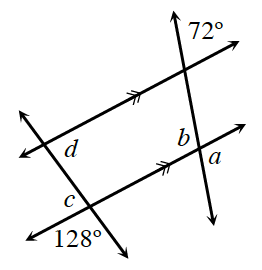### Home > INT1 > Chapter 3 > Lesson 3.3.3 > Problem3-139

3-139.

Examine the diagram at right. Then use the information provided in the diagram to find the measures of angles $a, b, c,$ and $d$. For each angle, name the relationship that helped justify your conclusion. For example, did you use vertical angles? If not, what type of angle did you use?

Review the Math Notes box in Lesson 1.2.1.